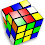## Saturday, June 07, 2008

### Guess this Social Security Number

A certain Social Security Number has the following qualities:
• It uses each of the digits 1 to 9 exactly once (with no zero).
• The digits from 1 to 2 (inclusive) add up to 12.
• The digits from 2 to 3 (inclusive) add up to 23.
• The digits from 3 to 4 (inclusive) add up to 34.
• The digits from 4 to 5 (inclusive) add up to 45.
• The digit 3 is NOT next to a dash (XXX-XX-XXXX).
What is this unique Social Security Number?

1.The answer is the product of the following primes:

3 x 3 x 3 x 5 x 3503099

2.Blaine, I assume that when you talk about the digits from 4 to 5 inclusive, a number like xyz54abcd encompasses all of the digits, i.e., you count from L to R only??

3.Don, under your interpretation is the solution unique?

4.Don, consider 1 and 2 the end points of a sequence of digits (e.g. 2451). That sequence adds up to 12. If you can arrange all 9 digits to meet the criteria, you've found the number.

5.Blaine, I guess that helps. By your problem definition, 4 and 5 are the end places of the SSN in question, as all 9 digits add up to 45. I found a sequence that fit 3 of the 4 criteria, but did not limit the placement of 4 and 5 as your reply did.

6.Here goes:

If I pick 4 as the first digit and 5 as the last digit:

4xx-xx-xxx5

4xx-xx-x365 fits the 34 clue

472-91-8365 then fits the remainder clues.

7.You got it! My logic was similar:

As you noted, 1+2+3+...+7+8+9 = 45, so the 4 and the 5 will have to be at the ends with a total of 36 between them, though at this point order could go either way. I'll use: 4 {36} 5

The numbers between 4 and 3 will add up to 34, which means 27 between them. What is left is 6.
4 {27} 3 {6} 5

The 2 must go closer to 4 in order for the sum of 23 to work. That puts 18 between them with 7 on the other side of 2.

4 {7} 2 {18} 3 {6} 5

And the 1 must go closer to the 3 for its sum to work: That puts 9 to the left and 8 to the right.
4 {7} 2 {9} 1 {8} 3 {6} 5

Technically there are two possible answers (472-91-8365 or 653-81-9279) but the puzzle also said that 3 is not adjacent to a dash so that eliminates the second answer.

8.